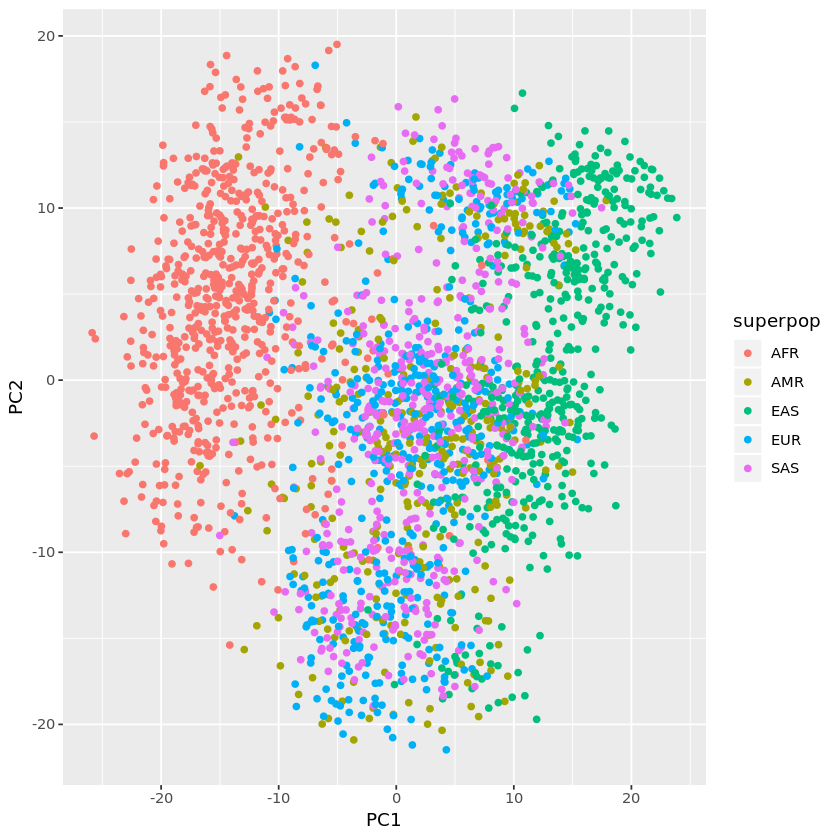# Population stratification illustration using slicing of remote VCF with Bioconductor¶

We will acquire a modest collection of SNP calls from chr17, and project the genotype configurations via principal components to expose population substructure.

## Create references to the EBI VCF repository¶

Bioconductor’s ldblock package includes utilities for working with collections of VCF, typically decomposed by chromosome.

In :
library(BiocManager)
inplist = rownames(installed.packages())
if (!("snpStats" %in% inplist)) install("snpStats")
if (!("ldblock" %in% inplist)) install("vjcitn/ldblock")
if (!("terravar" %in% inplist)) install("vjcitn/terravar")

In :
library(ldblock)
library(GenomicFiles)
library(VariantAnnotation)


## Read a slice of VCF corresponding to a region of chr17¶

EBI has updated 1000 genomes calls. We will interrogate a tabix-indexed VCF for chr17 via HTTP. An object that organizes multiple chromosomes is created using stack1kg from the ldblock package.

In :
st = stack1kg("17")


We use a ScanVcfParam to define a slice of genome.

In :
sp = ScanVcfParam(geno="GT",
which=GRanges("17", IRanges(32e6,33.75e6)))


readVcfStack produces a CollapsedVCF instance in memory. See the documentation for the VariantAnnotation package for details on this data structure.

In :
myread

class: CollapsedVCF
dim: 49194 2548
rowRanges(vcf):
GRanges with 5 metadata columns: paramRangeID, REF, ALT, QUAL, FILTER
info(vcf):
DataFrame with 12 columns: AF, AC, NS, AN, EAS_AF, EUR_AF, AFR_AF, AMR_AF,...
Number Type    Description
AF        A      Float   Estimated allele frequency in the range (0,1)
AC        A      Integer Total number of alternate alleles in called geno...
NS        1      Integer Number of samples with data
AN        1      Integer Total number of alleles in called genotypes
EAS_AF    A      Float   Allele frequency in the EAS populations calculat...
EUR_AF    A      Float   Allele frequency in the EUR populations calculat...
AFR_AF    A      Float   Allele frequency in the AFR populations calculat...
AMR_AF    A      Float   Allele frequency in the AMR populations calculat...
SAS_AF    A      Float   Allele frequency in the SAS populations calculat...
VT        .      String  indicates what type of variant the line represents
EX_TARGET 0      Flag    indicates whether a variant is within the exon p...
geno(vcf):
SimpleList of length 1: GT
Number Type   Description
GT 1      String Phased Genotype

## Transform the VCF content to rare allele counts¶

We use tools in David Clayton’s snpStats package to achieve a compact representation of (possibly uncertain) genotype calls. This enables us to filter to SNP with MAF exceeding a given threshold, and, later, to compute a PCA.

In :
library(snpStats)
## non-single nucleotide variations are set to NA
cs = col.summary(mymat[])
sum(cs[,"MAF"]>.1, na.rm=TRUE)

non-single nucleotide variations are set to NA

2909

## Build a matrix of B-allele counts¶

We'll use SNP with MAF exceeding 10%, and build the sample x SNP matrix.

In :
kpsnp = which(cs[,"MAF"]>.1)
cmat = matrix(0, nr=nrow(mymat[]), nc=length(kpsnp))
for (i in seq_len(length(kpsnp))) {
cmat[,i] = as(mymat[][,kpsnp[i]], "numeric")
}
rownames(cmat) = rownames(mymat[])


## Compute principal components and plot¶

In :
pp = prcomp(cmat)

In :
dim(pp$x)  1. 2548 2. 2548 In : library(ggplot2) library(terravar) data("geog_1kg") rownames(geog_1kg) = geog_1kg[,1] newdf = data.frame(pp$x[,1:4], pop=geog_1kg[rownames(pp$x), "Population"], superpop = geog_1kg[rownames(pp$x), "superpop"])

In :
ggplot(newdf, aes(x=PC1, y=PC2, colour=superpop)) + geom_point()